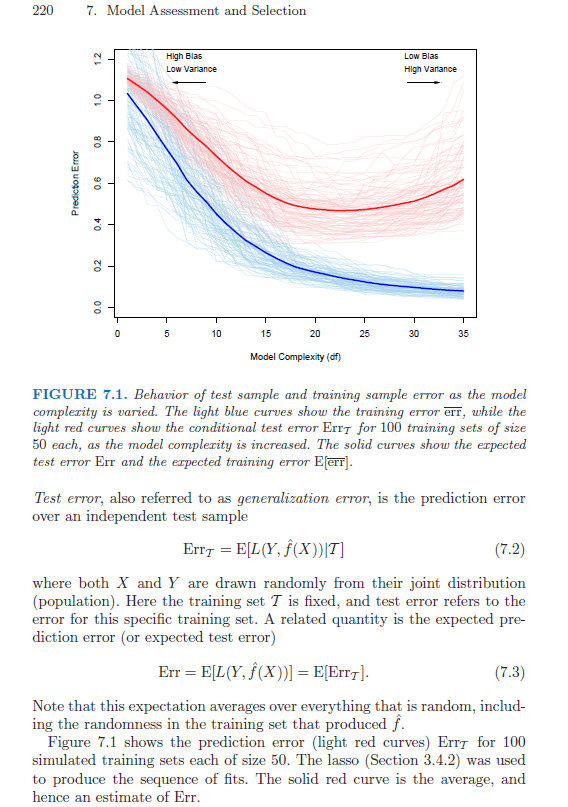# #StackBounty: #regression #prediction #simulation Using test data sets in simulation study

### Bounty: 50

I’d like to know the correct way to simulate test data for a simulation study. For simplicity, suppose that I want to test a linear regression model. Chapter 7 of ESL explains that the average test error is equal to the expected prediction error, where the average is over the training data.

$$text{Err}{mathcal{T}}$$ is the test error for a specific training set $$mathcal{T}$$. They have an example stating that $$text{Err}$${mathcal{T}}\$ was calculated for 100 simulated training sets. $$sum_{mathcal{T}=1}^{100}text{Err}{mathcal{T}}/100$$ is then an estimate of the expected prediction error $$text{Err} = E(text{Err}$${mathcal{T}})\$.

Page 220 of ELS:My question is: must 100 test sets be simulated for the calculation or is each $$text{Err}_{mathcal{T}}$$ calculated using the same test set?

Here is some R code to demonstrate:

``````library(MASS)
n = 50
m = 200
b0 = 0.5
b = c(1,2,0,0)
rho = 0.5
sigma = 1
iter = 100
p = length(b)
r = matrix(rho, p, p); diag(r) = 1

# test set option A
# x.new = mvrnorm(m, rep(0,p), r)
# y.new = b0 + x.new %*% b + rnorm(m, 0, sigma)

err.t = rep(0, iter)
for (i in 1:iter) {

# training set
x = mvrnorm(n, rep(0,p), r)
y = b0 + x %*% b + rnorm(n, 0, sigma)

# test set option B
x.new = mvrnorm(m, rep(0,p), r)
y.new = b0 + x.new %*% b + rnorm(m, 0, sigma)

mod = lm(y ~ x)
pred = predict(mod, x.new, type="response")
err.t[i] = sum((y.new - pred)^2)/m
}
err = mean(err.t)
``````

Should the test set be generated outside the loop as in option A, or inside the loop as in option B?

The same question goes for a validation set. Suppose I was fitting a LASSO model and want to use a separate data set for model selection, i.e. choose the best tuning parameter, should the validation set be outside or inside the loop?

Get this bounty!!!

This site uses Akismet to reduce spam. Learn how your comment data is processed.